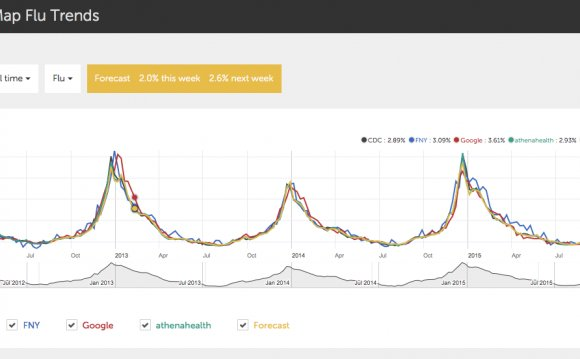# European Journal of Applied Mathematics

September 8, 2022A1 Association EURATOM-MEC Department of Theoretical Physics, Physics Faculty, University of Craiova, Str.A.I.Cuza 13, Craiova-200585, Romania email:

a2 Department of Mathematics King's College, The Strand, London WC2R 2LS, England, UK email:

Abstract

In both modern stochastic analysis and more traditional probability and statistics, one way of characterizing a static or dynamic probability distribution is through its quantile function. This paper is focused on obtaining a direct understanding of this function via the classical approach of establishing and then solving differential equations for the function. We establish ordinary differential equations and power series for the quantile functions of several common distributions. We then develop the partial differential equation for the evolution of the quantile function associated with the solution of a class of stochastic differential equations, by a transformation of the Fokker–Planck equation. We are able to utilize the static formulation to provide elementary time-dependent and equilibrium solutions.

Such a direct understanding is important because quantile functions find important uses in the simulation of physical and financial systems. The simplest way of simulating any non-uniform random variable is by applying its quantile function to uniform deviates. Modern methods of Monte–Carlo simulation, techniques based on low-discrepancy sequences and copula methods all call for the use of quantile functions of marginal distributions. We provide web resources for prototype implementations in computer code. These implementations may variously be used directly in live sampling models or in a high-precision benchmarking mode for developing fast rational approximations also for use in simulation.